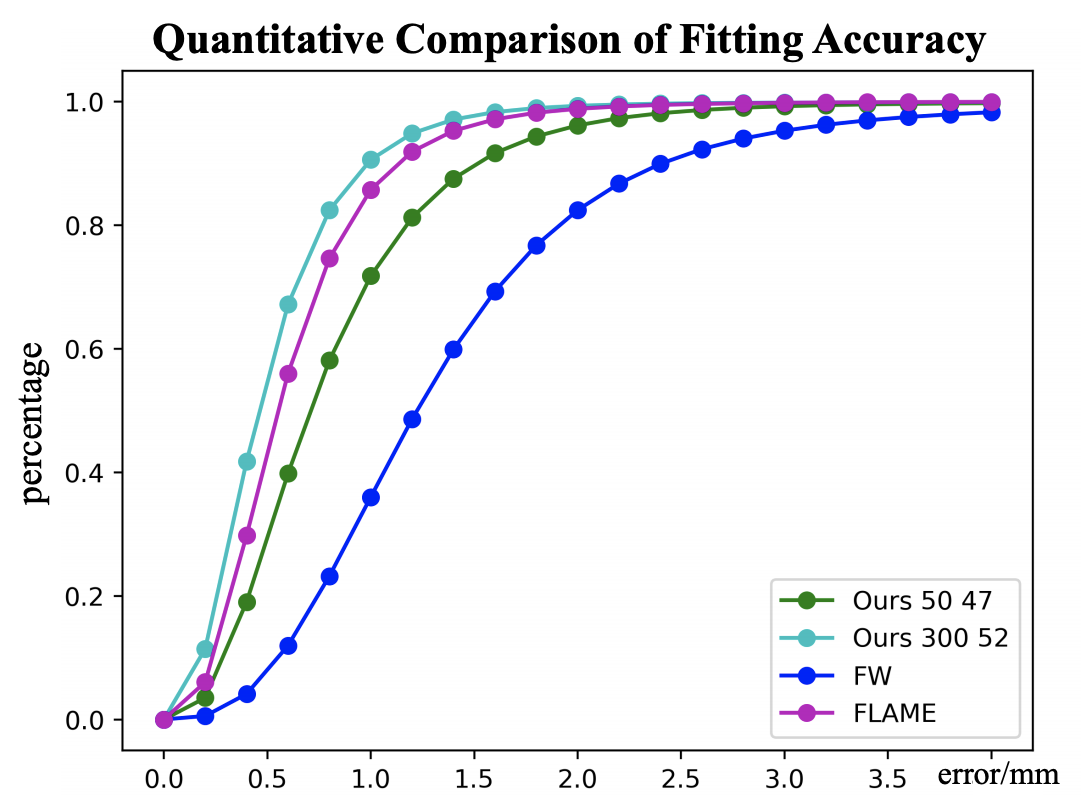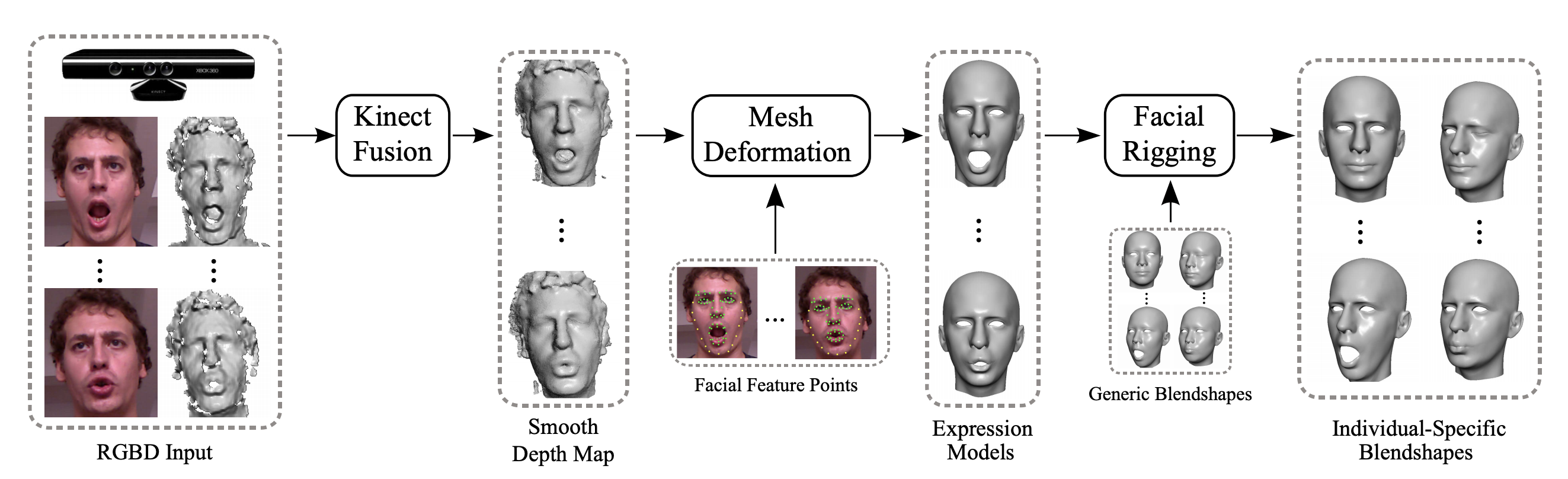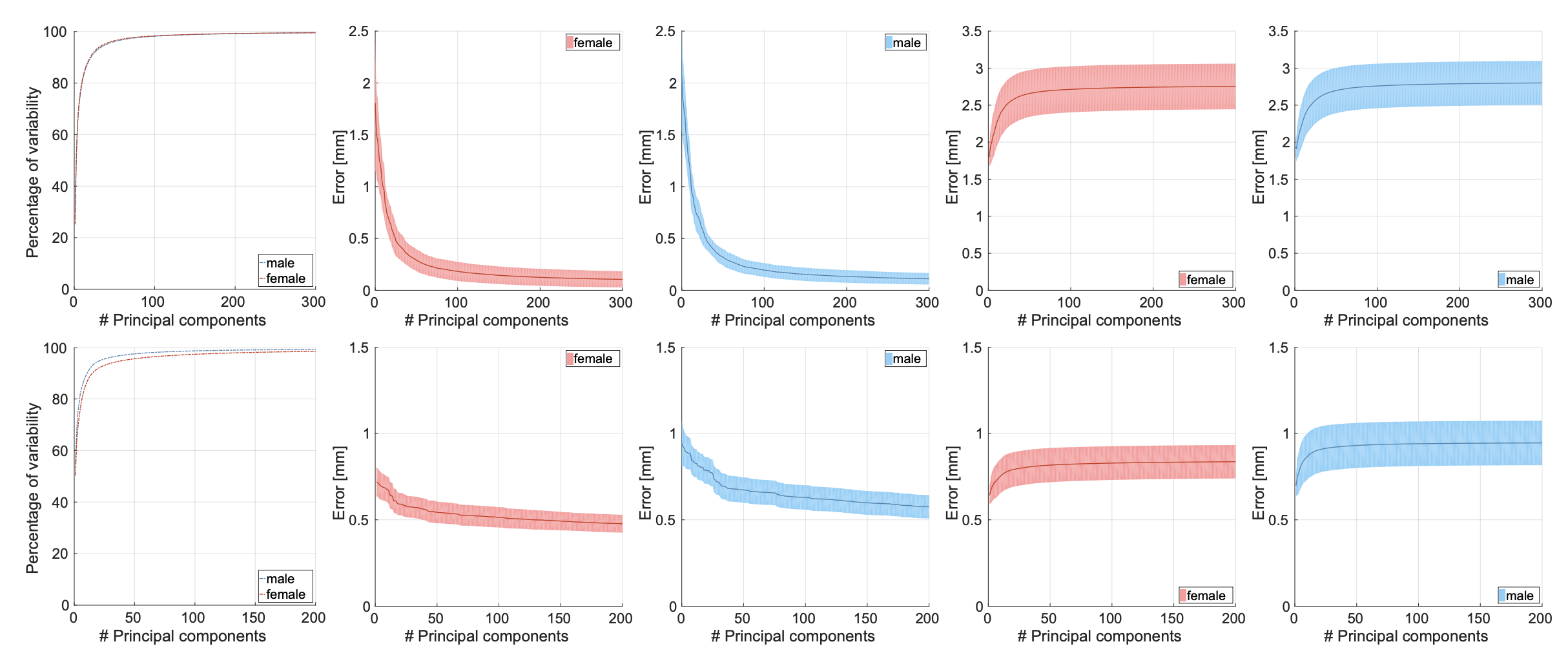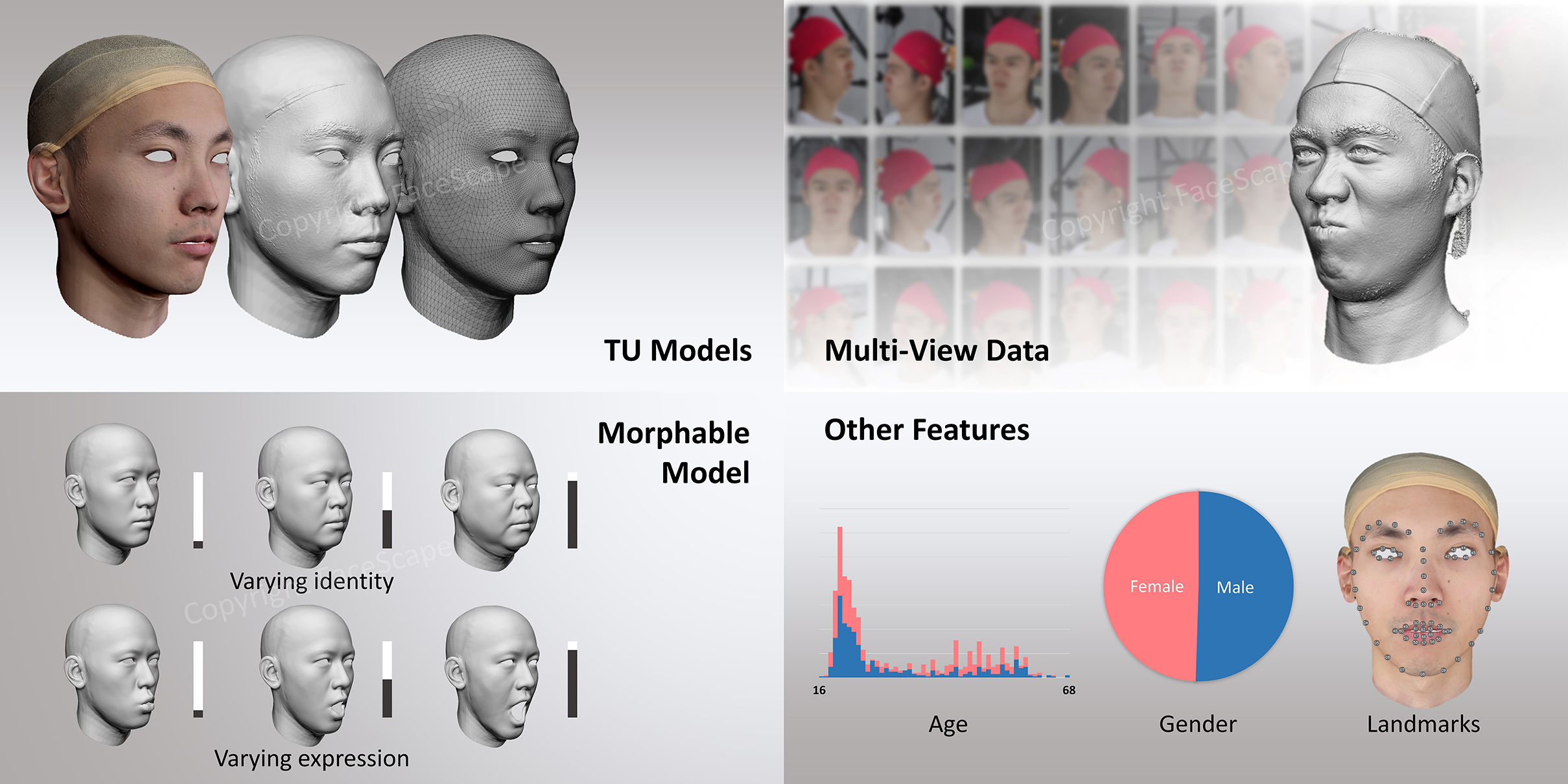# 3D Morphorble Model

This notebooks describes the models which could represent animatable 3D face mesh, which is usually referred 3D morphorble model (3DMM).# FaceWareHouse

FaceWareHouse is a database of 3D facial expressions for visual computing applications. Some highlights:

• learned from data captured from Kinect includes 159 subjects and 20 expressins each. The quality and resolution of Kinect depth camea is at millimeter level;
• bilinear model with identity and expression on different axis $$V=C_r\times W_{id}\times W_{exp}$$, with $$W_{id}\in\mathbb{R}^{150}$$ and $$W_{exp}\in\mathbb{R}^{47}$$;# FLAME

FLAME parametrizes the 3D face mesh by identity $$\beta\in\mathbb{R}^{100}$$, expression $$\psi\in\mathbb{R}^{50}$$ and head pose $$\theta\in\mathbb{R}^{15}$$). Some highlights of FLAME:

• learned from 33000 3D scans of several hertergenous dataset including about 3800 identities, 8000 scans for poses and 69000 frames for expressions;
• strictly linear model for identity, expression and pose;
• the idenity and expression basis are orthornomal and computed from data. The number of basis are configurable;
• pose contains global pose, neck pose, jaw pose and two pupil poses;
• FLAME also supports different rendering to output the 2D image.

FLAME could be written as:

$M(\beta,\theta,\psi)=W(T+B_S(\beta,S)+B_p(\theta,P)+B_E(\psi,E),J(\beta),\theta)$This figure shows the coverage of variability given different number of basis.# FaceScape

FaceScape provides large-scale high-quality 3D face datasets, parametric models, docs and toolkits about 3D face related technology. It is published in CVPR 2020 and code is available in github. FaceScape utilizes a bilinear model instead of linear model in FLAME.Some highlights of FaceScape:

• learned from 18760 scans from 938 subjects and 20 expressions each;
• bilinear model with identity and expression on different axis $$V=C_r\times W_{id}\times W_{exp}$$, with $$W_{id}\in\mathbb{R}^{938}$$ and $$W_{exp}\in\mathbb{R}^{52}$$;
• captured with high quality multi-view systemconsists of 68 DSLR cameras, 30 of which capture 8K images focusing on front side, and the other cameras capture 4K level images for the side part.
Written on April 24, 2021